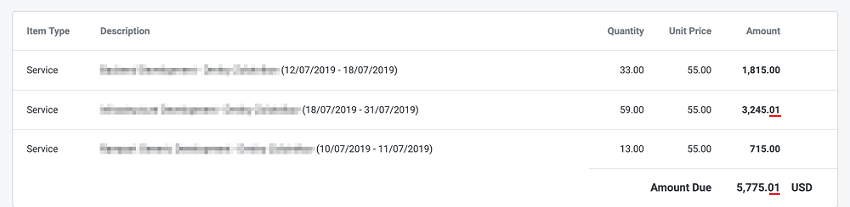# Why is there an additional cent in the total Amount?

What you see in the invoice is a result of multiple operations being concurrently executed.

The Amount value is not calculated by multiplying Quantity and Unit Price. It is calculated for each time entry separately (since in most cases there is more than one rate), and then it is summed up.

And Unit Price is calculated at the very end as an average value of dividing Amount by Quantity.

Besides that Quantity is a decimal number that is calculated according to the formula Q = T(min) / 60. Here, in general, there is no way to avoid rounding, for example, a 10-minute time entry is equal to 0.1666666667 h (hour).